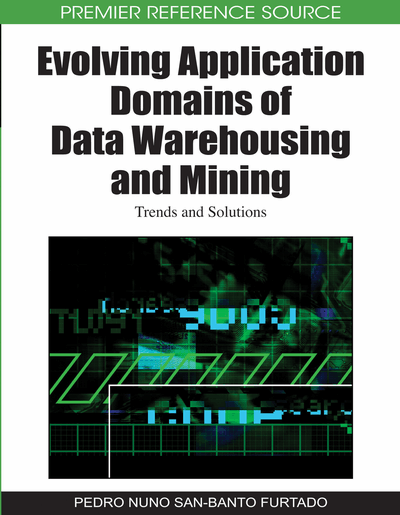# A Multidimensional Model for Correct Aggregation of Geographic Measures

Sandro Bimonte (Cemagref and UR TSCF, France), Marlène Villanova-Oliver (Laboratoire d’Informatique de Grenoble, France) and Jerome Gensel (Laboratoire d’Informatique de Grenoble, France)
DOI: 10.4018/978-1-60566-816-1.ch008
Available
\$37.50
No Current Special Offers

## Abstract

Spatial OLAP refers to the integration of spatial data in multidimensional applications at physical, logical and conceptual levels. The multidimensional aggregation of geographic objects (geographic measures) exhibits theoretical and implementation problems. In this chapter, the authors present a panorama of aggregation issues in multidimensional, geostatistic, GIS and Spatial OLAP models. Then, they illustrate how overlapping geometries and dependency of spatial and alphanumeric aggregation are necessary for correctly aggregating geographic measures. Consequently, they present an extension of the logical multidimensional model GeoCube (Bimonte et al., 2006) to deal with these issues.
Chapter Preview
Top

## Introduction

A Data Warehouse (DW) is a centralized repository of data acquired from external data sources and organized following the multidimensional model (Kimball, 1996) in order to be analyzed by On-Line Analytical Processing (OLAP) systems. Multidimensional models rely on the concepts of facts and dimensions. Facts are described by values called measures. Dimensions, structured in hierarchies, permit to analyze facts according to different analysis axes and at different levels of detail. An instance of a dimension is a set of members organized according to the hierarchies. An instance of the conceptual model is represented by a hypercube whose axes are the dimension members at the finest levels. Each cell of a hypercube contains the value of the detailed measure. This basic cube (also called facts table) is then enhanced with cells that contain aggregated values of the measures for each combination of higher level’s members. Aggregation operators applied on the measures must be specified in the conceptual model and depend on the semantics of the application. The classical functions used to aggregate numeric measures are the standard SQL operations “COUNT”, “SUM”, “MIN”, “MAX” and “AVG”. The multidimensional model allows pre-computation and fast access to summarized data in support of multidimensional analysis through OLAP operators which permit to explore the hypercube. Drill operators (Roll-Up and Drill-Down) permit to navigate in the dimensions hierarchies aggregating measures. Cutting operators (Slice and Dice) select and project a part of the hypercube. The multidimensional model and OLAP operators have been formalized in some logical models (Abello et al., 2006) as a support to correct aggregation of measures which plays a central role in multidimensional analysis (Pedersen et al., 2001). They define constraints on the aggregation functions in compliance with the semantics of the measure and explicit the dimensions that can be used in the multidimensional queries.

Most of 80% of transactional data contain spatial information, which represents the form and the location on the earth surface of real world objects (Franklin, 1992). The heterogeneity of physical spaces and the strong spatial correlation of thematic data (Anselin, 1989) are not taken into account into multidimensional models. Then, a new kind of systems have been developed, which intended to integrate the spatial component of the geographic information into multidimensional analysis: Spatial OLAP (SOLAP) (Bédard et al., 2001). Spatial OLAP allows decision-makers to explore, analyze and understand huge volume of geo-spatial datasets, in order to discover unknown and hidden knowledge, patterns and relations. This useful information can help spatial analysts and decision-makers to validate and reformulate decisional hypothesis, and to guide their spatial decision making processes. SOLAP technologies have been usefully applied in several domains: geo-marketing, urban, health, environment, crisis management, etc. (Bédard et al., 2001) as they allow non-computer science users to exploit databases, statistical analysis and spatial analysis tools without mastering complex query languages and Geographic Information Systems functionalities, and understanding underlying complex spatial datasets. SOLAP redefines main multidimensional concepts: spatial dimensions, spatial measures and spatial aggregation functions. In this approach, spatial measures are not numerical values, but spatial objects (geometries) which are aggregated using spatial aggregation functions (union, intersection, etc.) (Shekar et al., 2001). As shown in this work, SOLAP models only partially support dependency of spatial and numerical values, which can lead to wrong aggregation of spatial and numerical measures (geographic measures).

In this paper, we identify a three-step aggregation process for the correct aggregation of geographic measures, and we formalize it by providing an extension of the logical multidimensional model, GeoCube (Bimonte et al., 2006). The model provides a set of rules to ensure the valid aggregation of geographic measures.

## Complete Chapter List

Search this Book:
Reset